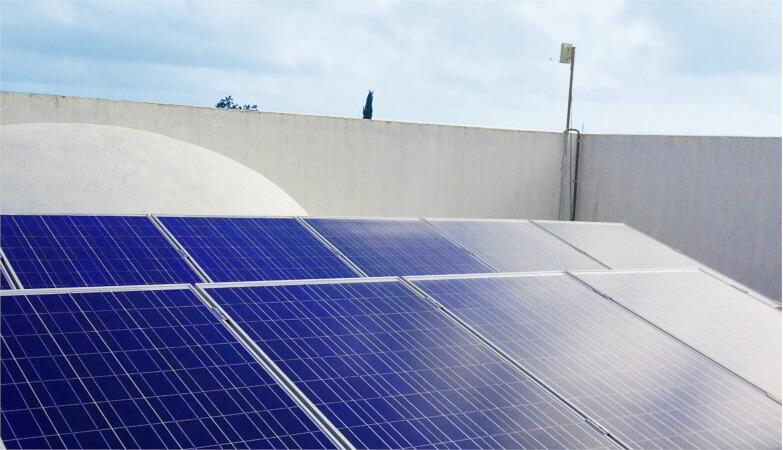Technical Article

# Selecting and Sizing Solar System Components

April 20, 2023 by Simon Mugo

## This article explains how to design solar power systems with a focus on calculating energy requirements and sizing solar panels, batteries, inverters, and charger controllers.

The world is fast moving toward 100% green and clean energy consumption. Most countries are working hard to use green energy to preserve and protect the environment from pollution and global warming. This goal has triggered most countries to look for alternative ways to meet energy demands. One such way is by harvesting energy from the sun.##### Solar panels. Image used courtesy of Pixabay

The introduction of solar panels has helped, including monocrystalline, polycrystalline, and thin film. But solar panels alone are not enough, and storage like batteries is needed for the power generated by the solar panels. A complete solar system also needs a voltage inverter and charge controller. This article will focus on these solar power system components and how to select and size them to meet energy needs.

### Solar System Components

A complete solar power system is made of solar panels, power inverters–specifically DC to AC–charger controllers, and backup batteries.

#### Solar Panels

Solar panels are the most common component. They are also referred to as photovoltaic panels. Solar panels are composed of many solar cells, and every solar system is built up of many technically arranged solar panels, referred to as the solar array.

Most solar panels are installed on building roofs and, in some cases, mounted on car roofs as movable off-grid panel components or grounded based on the need. They are set at an angle toward the sun to absorb the most solar energy throughout the day.

#### Solar Batteries

Solar batteries are an optional component when setting up a solar power system, but home solar systems should have them to store energy. During the day, the battery will accumulate power and store it to use at night. More energy storage requires more batteries–referred to as the battery bank. Batteries come in many types, including lead-acid, flow, lithium-ion, and nickel-cadmium.

#### Charge Controller

The charge controller manages the power flow from the solar panel to the connected battery. Without a battery connected to the system, charge controllers are not required. They work by ensuring the battery charges to the maximum level to enhance its longevity. Two types exist: maximum power point tracking and pulse with modulation.

#### Solar Power Inverters

Solar power inverters are crucial components in converting DC-generated energy into AC.

### Solar System Component Selection and Sizing

• Step 2: Select the solar panel
• Step 3: Select the battery size
• Step 4: Select the inverter
• Step 5: Select the charger controller

#### Step 1: Calculate the Electrical Load

The table below assumes a simple loading system, but this calculation method should work for large solar power systems of over 1 MW of power generation.

##### Table 1. Electrical Load Calculations
 S. NO LOAD NAME LOAD POWER(W) NO. OF LOAD TOTAL LOAD(W) OPERATION HOURS(h) TOTAL ENERGY(Wh) LED light 10 5 50 6 300 Tube Light 18 4 72 5 360 Television 40 2 80 10 800 Ceiling Fan 50 4 200 8 1600 Refrigerator 175 1 175 10 1750 TOTAL POWER 577 TOTAL ENERGY 4810

Therefore, the total electrical load calculation that our system will drive is 4810 Wh, and the total power is 577 W.

#### Step 2: Select the Solar Panel

Total Power Required per Day = 557 W

Total Energy that is Required per Day = 4810 Wh

$Power\,Required\,from\,the\,Solar\,PV\,(Wh)=\frac{Total\,Energy\,Required\,from\,Solar\,PV\,(Wh)}{Effective\,Sunshine\times System\,Efficiency(\eta_{s})}$

Effective Sunshine for California, USA = approximately 6.25 h

$System\,Efficient=\eta_{B}\cdot\eta_{i}\cdot\eta_{oth}$

Where

$\eta_{B}=Efficiency\,of\,the\,Battery\,assumed\,to\,be\,90\%$

$\eta_{i}=Efficiency\,of\,the\,Inverter\,assumed\,to\,be\,95\%$

$\eta_{oth}=Other\,Components\,such\,as\,cables\,Efficiency\,is\,assumed\,to\,be\,85\%$

Therefore

System Efficiency = 0.9 × 0.95 × 0.85 = 0.73

$Power\,Required\,from\,the\,Solar\,PV\,(Wh)=\frac{4810}{6.25\times0.73}=1054\,W$

Therefore, the power required from solar panels is approximately 1200 watts.

$The\,Number\,of\,Solar\,Panels\,Required=\frac{Power\,Required\,for\,Solar\,Panel\,(Wh)}{Output\,Power\,of\,a\,Single\,Solar\,Panel}$

Each solar panel is rated at 200 W.

Therefore

$The\,Number\,of\,Solar\,Panels\,Required=\frac{1200}{200}=6\,Panels$

Select six solar panels each rated at 200 W to meet the energy demand of the home.

#### Step 3: Battery Selection

Total Power Required per Day = 557 W

Total Energy Required per Day = 4810 Wh

$Total\,Required\,Battery\,Capacity(AH)=\frac{Total\,Backup\,Energy\,Per\,Day}{Battery\,Voltage\times Depth\,of\,Discharge\times Battery\,Efficiency}$

Where

Battery Voltage = 12 V

Depth of Discharge = 85%

Battery Efficiency = 85%

Therefore

$Total\,Required\,Battery\,Capacity(AH)\frac{4810}{12\times0.85\times0.85}=555AH$

$Number\,of\,Batteries\,Required = \frac{Total\,Required\,Battery\,Capacity\times Backup\,Days}{Required\,AH\,of\,Single\,Battery}$

Let the required AH of each battery be 200 AH.

Therefore

$Number\,of\,Batteries\,Required = \frac{555×1}{200}=2.775 \cong 3\,Batteries$

The battery selection should give an AH of above 555 and result in 3 batteries, each rated at 200 AH.

#### Step 4: Inverter Selection

Total Power Required per Day = 577 W

$Required\,Inverter\,Power = \frac{Total\,Power\,Required\,Per\,Day\times Safety\,Factor}{Inverter\,Efficiency}$

Inverter Efficiency = 95%

According to NEC, the inverter safety factor should be between 25% and 30%.

The inverter safety factor is 25% high.

$Required\,Inverter\,Power=\frac{577\times1.25}{0.95}=759 W \cong 1000\,W$

The required inverter power is approximately 1000 W.

#### Step 5: Charger Controller Selection

Charge controller sizing can be determined using the battery bank voltage and total solar panel power.

Therefore

$Charger\,Controller\,Current\,Rating=\frac{Total\,Solar\,Panel\,Power\,(W)}{Battery\,Bank\,Voltage\,(V)}=\frac{1200}{12}=100\,A$

### Takeaways of Selecting Solar System Components

• A solar power system comprises solar panels, batteries, inverters, and charge controllers.
• Solar panels are the most common components in the solar energy system used in harvesting energy from the sun.
• Solar batteries are used to store energy in a solar system where they accumulate energy during the day.
• The charge controller manages the power flow from the solar panels to the connected batteries.
• Solar power inverters convert DC power from the battery into AC power to be consumed by several pieces of equipment in the home.
• Five steps are involved in the selecting and sizing of the solar energy system: calculating the electrical load of the whole home and selecting the solar panels, battery size, inverter, and charger controller.